# Study of Hydrodynamic Forces on Multiple Cylinders in Waves Introduction In the recent years, there have been increase in demand of production in the offshore industry. The wide market scale of offshore structure business in oil and gas production attracts interest for investors. Offshore structures are stationary structures located in the marine environment for production of natural resources such as wind turbine for generating electricity, FPSO (Floating Production Storage Offload) for crude oil extraction and drilling for drillships. These offshore structures can be either fixed or freely floating. Floating offshore structures/platforms will be using mooring system or dynamic positioning method to float and hold its position in place. Many challenges are faced to withstand the position of the offshore structures due to seakeeping and wave load problems. As technology advance over the years, new implications and investigations are being carried out for offshore structures to to deal with seakeeping and wave load problems. The implication of hydrodynamic force acting on an offshore structure became important due to various wave loads and interactions might affect the results of hydrodynamic forces greatly. The hydrodynamic forces determine how structures float with varying wave frequencies, wave height and etc. Large offshore structures such as semi-submersibles, offshore wind turbines and SPAR are often being investigated to improve their efficiencies by changing their shapes or dimensions. Large offshore cylindrical structures would affect hydrodynamic results due to their wave diffraction and radiation forces. Therefore, in this literature review, investigations of hydrodynamic forces on multiple cylinders are being studied. In order to understand how the hydrodynamic results vary, floating bodies with varying distance are being placed in ideal fluid, where hydrodynamic interactions will cause the influence by the scattered waves and the wave load will differ greatly by the presence of the neighbouring cylinders. Thus, to obtain accurate results, these hydrodynamic interactions are investigated from theoretical predictions, software simulation analysis and model testing. The development of computer with memory capacity and computational speed increases with the advance in technology. The use of these software simulation analysis helps to analyse the wave interactions between multiple floating bodies in an ideal fluid. The software is dependant on the development of hydrodynamic theories. By using linear wave potential theory, the diffraction and radiation forces could be calculated. Hydrodynamic Interaction phenomena Studies of hydrodynamic interactions of multiple bodies have been on going over the past few decades. Hydrodynamic interaction created are due to wave actions the presence of neighbouring structures. A free floating body may experience six DOF (Degree of Freedom) due to surrounding condition, where three are translations (Surge, sway and heave) and three are rotations (Roll, pitch and yaw). Vertical and longitudinal motions are the primary motions which are often experience by offshore structures. The interaction phenomena is due to diffraction and radiation forces acting on the structures and forces created by the structures. The velocity components and the motion of each body can be obtain by the integration of equation of motion. The interaction phenomena between two or more floating structures plays important role on the performance of floating structure systems. It depends on the sepetration distance between the floating bodies and also on the direction of flow with respect to the centerline of two floating bodies. This phenomena can be investigated by using both theoretical and experimentally(Model Test). For experimental, using a full scale model tests are desirable but there are limitations due to controlled conditions and the high cost of production. Therefore, the need of associated computation tools for analysing these hydrodynamic forces to attain fast and accurate results. The complexity of multiple bodies solution increases with the amount of elements.(Research_3) Thus, the use of numerical method is highly recommended in this studies. http://www.ias.ac.in/sadhana/Pdf2004Dec/Pe1236.pdf  http://www.dtic.mil/dtic/tr/fulltext/u2/a254627.pdf  Potential Theory The knowledge of motion and wave induced forces of offshore mainly involves the structural design and its operation. Offshore structures in sea environment usually encounter environmental loads such as gravity, waves, wind and current. These are the basic information needed to evaluate the interaction of structures through the use of potential theory.(G. Moe, 1996) Potential theory can use to find out the general aspect of fluid flow problems in conjunction with Laplace equation. To satisfy the solution of the Laplace equations, boundary conditions of the fluid are set. Hydrodynamic forces in waves consist of radiation and diffraction forces where velocity potential is the key component to satisfy the Laplace equation. Radiation and Diffraction Problem Hydrodynamic forces is made up of diffraction and radiation forces. These forces includes the 6 degree of freedom that arises from the interaction of linear waves against any floating structure. In addition, these forces will provide information of hydrodynamic properties of floating structures, such as damping coefficient,added mass and wave excitation forces. The fluid must be assumed to be inviscid, incompressible and irrotational. Currently, there have been numerous theoretical and numerical studies on these problems. As the technology advances, researchers have obtained significant results on the phenomena, which made the study for these problems, such as structure and wave interactions, stability of floating structures aon going research. Diffraction Force Diffraction force is the pressure forces acting around the each structure, but due to multiple bodies and interaction of several bodies, the scattering will affect the results of incident wave. For free surface problems with the Laplace equation as the governing equation, a first order or a second-order boundary condition can be obtain using a linearized free surface boundary condition Radiation Force Boundary Conditions& Governing Equation The motion response of a structure will cause the fluid to oscillate, hence creating pressure fields in the fluid. In order to capture the motion response and the surrounding pressure fields, velocity potential will be imposed. In addition, there are boundary conditions critition to be met. Drag forces are not being considered in the radiation and diffraction problems as it only occurs in slender body phenomena. With the assumption of having the structure placing in an ideal fluid with zero forward speed. In an ideal fluid condition, the fluid is irrotational and incompressible. The radiation condition should also be satisfied as it is to ensure that the waves propagate away from the structure. From the potential flow theory, it is made known that the velocity potential must satisfy the Laplace equations. Thus, the 3 boundary conditions mention below must be satisfied. Body surface The normal component of the fluid velocity is equal to the normal component of the heave force velocity Seafloor The normal component of the fluid velocity equal to zero Water surface The fluid pressure is equal to the atmospheric pressure and fluid particles will always remain on the free-surface. Pressure will be constant across the free surface.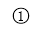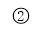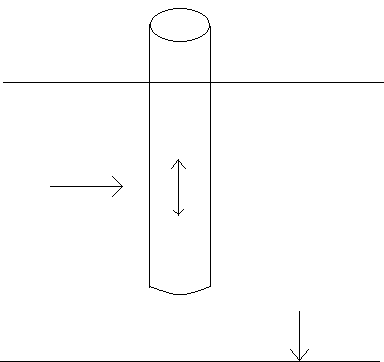These equations mention below will show the governing equation imposed on radiation and diffraction problems.(Page 44) The velocity potential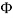, along each component(x, y and z) satisfies the Laplace equation in the fluid domain,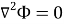(1) The free-surface condition,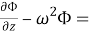0 on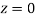(2) Boundary condition on the sea floor,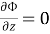for finite water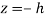(3)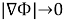for infinite water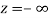(4) Boundary condition on body surface,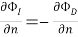(5) Where k is depends on the motion (six DOF) that the body surface is expose to and nis the unit vector normal to the body surface. Solving Boundary Value Problem The boundary conditions are all set in conjunction with velocity potential. The expression of linear hydrodynamic forces is derived from direct integration of the fluid pressure over the boundary of a body. By using the Green’s theorem, part of the forces can be obtained with the solving the scattering potential. Defining the field point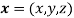at any point and the source point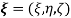for circulation, rotation and vorticity, the Green funtion will be presented in the form,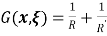when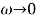(6)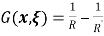when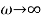(7) Where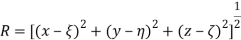(8)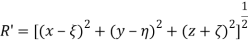(9) Equation 7 expresses the source potential as a summation of a source in the finite fluid and an image source above the free surface condition where. This shows that there will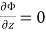at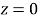. Equation 8 expresses the source potential is a summation of a source in infinite fluid and an image sink above free surface when. This satisfy the free surface condition where velocity potential equal zero on. Therefore, the solution of the velocity potential for a problem (e.g. force heave) can be written as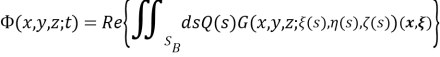(10) This source function is given for infinite water depth problem where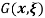is called the Green function. By using the Bernoulli’s equation, the total pressure could be fully derived with the use of velocity potential and the body motion amplitudes. Therefore, linear forces and moments are obtain from,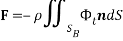(11)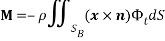(12) We can consider component potentials such that the summation of hydrodynamic forces and moments are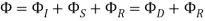Where it is split into two components: the wave excitation force due to diffraction and forces due to radiation force(In terms of added mass and damping coefficients). Given we want to find the force at mode j. The linear forces, also known as the first order wave excitation force, the force for mode j is denote as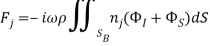From Green’s theorem, applying it to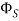and a component of the radiation potential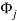, we have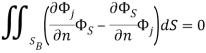It is show that those integrals on the seabed, over the free surface and at the far field disappeared due to the boundary conditions forandon these surfaces. Therefore, upon substituting the equation of boundary conditions, the Haskind relation follows in the form of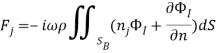By using the equation of motion, the diffraction and radiation problem will be solved. Calculation of Hydrodynamic forces The boundary value problem is solve, therefore the forces and moments can be evaluate. Panel method is use to solve the boundary value problem which is based on potential theory. Assumptions such that the wave amplitudes of the fluid is small as compared to the cross-sectional dimensions of the body are made. The panelling of the structure is done by plane quadrilateral elements in three dimensional problems. http://www.academia.edu/6937922/Computation_of_Ship_Hydrodynamic_Interaction_Forces_in_Restricted_Waters_using_Potential_Theory The solution procedure can be generalized to any modes of motion, where added mass and damping matrix could be found. Solving the diffraction problem can be done by using the boundary condition (equation 5) where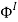is the incident wave potential. From these solutions, we are able to derive the wave excitation loads such as wave excitation forces (surge, sway, heave) and wave excitation moments (roll, pitch, yaw). Therefore, solving the equation of motion and calculating the flow details such as hydrodynamic forces, by using the expression for velocity potential in terms of a three-dimensional source distribution. Past Researches Overview WAMIT Wamit software is a diffraction/radiation panel program developed to analyse the linear interaction of surface waves with several different types of submerged and floating structures. For this analysis of interaction, WAMIT uses a three-dimensional panel method and also following some theoretical method. By using this software, it can help to define the conditions of the interactions such as, finite or infinite water depth, structures location on the free surface, mounted on the seabed or submerged and whether the structures are fixed or freely floating with the motion of six degree of freedom. The fluid flow is assumed to be ideal and time harmonic. WAMIT is able to deal with both first order and linearize analysis which includes second order forces. WAMIT is able to focus on the hydrodynamic interaction of multiple body with any restriction of condition of fluid environment. The use of integral equation obtained by Green’s theorem will help solve the diffraction and radiation velocity potentials on the body wetted surface. WAMIT will be able to evaluate the following quantities: Hydrostatic coefficients Added-mass and damping coefficients for all modes Wave excitation forces and moments using the Haskind relations, or directly by pressure integration from the solutions of diffraction or scattering problems. Motion amplitudes and phases for a freely floating body Forces restraining a body which is freely floating in some but not all modes Hydrodynamic pressure and fluid velocity on the body surface/fluid domain Free-surface elevation All component of the drift force and moment by momentum integration over a control surface Horizontal drift forces and mean yaw moment by momentum integration in the field All components of the drift force and moment by local pressure integration over body surface Drift force and moment in bidirectional waves Theoretical Method The method use by WAMIT will be a panel program designed to solve boundary-value problem for the interaction of waves phenomena with the pre-defining the bodies in finite or infinite water depth. Viscosity will not be considered. The time harmonic solutions are being solved for a given steady state incident wave field which is assumed to be represented by a superposition of a particular frequency components in the absence of the body. The boundary value problem will be used in conjunction with the integral equation using Green’s theorem. These integral equation will be solved by panel method for the unknown velocity potential or strength source of the body surface. These integral equations of theoretical method could be found in .

## Order a Unique Copy of this Paper

Essay Creek is an academic writing service provided to you by, a London-based company.

• Experience
Helping students successfully for 11 years.
• Confidentiality & Security
Be sure your information will be kept confidential due to our secure service.
• Quality & Reliability
8.5 out of 10 average quality score according to our customers' feedback. 97.45% of orders delivered on time.
• Versatility
478 active writers in 68 disciplines.
• 100% money back guarantee
You can always request a refund if you are not satisfied with the result.

Our team of writers is comprised of people with necessary academic writing skills and experience in various fields of study.

• Skilled writers only
We carefully choose writers to employ, paying attention to their skills and abilities.
• Competence
Your order will be assigned to a competent writer who specializes in your field of study.
• In-depth knowledge
Our writers know both peculiarities of academic writing and paper formatting rules.
• Motivation
We keep updated on results our writers show, motivating them to constantly improve their performance.